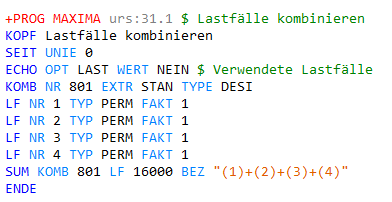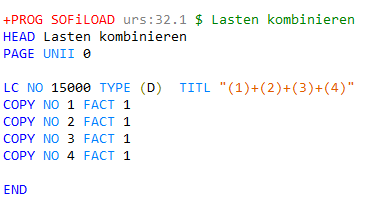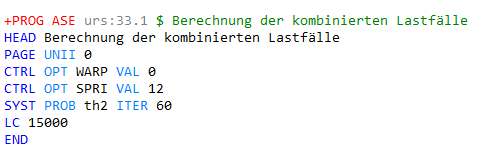# What is the difference to use define combinations and superpositioning with combine loads and analysis of combined loadsand

As we know, we have define combinations and superpositioning Module in SSD, else, we also have combine loads and analysis of combined loads Module in SSD. my question is what is the difference of above two sets? if i do steel frame structure analysis(like a steel frame factory) or solar support structure, which set should i use?

Hello

A detailed description of our SSD tasks can be found on our website.

We even have tutorials for the analysis of steel structures.

www.sofistik.de > Infocenter - Überblick > Dokumentation - Tutorials > Design of Steel Structures

Best regards
Frederik Höller

Yes i checked the tutorial. Per my understanding, if we only do “Steel Cross Sections Resistance”, above both have same effect. only in case when we do “Steel Buckling Resistance”, we need use “Combined Loads” and “Analysis of Combined Loads”, am i right?

So the differences are:

• superposistioning: calculates automatically the superposition of multiple available already calculated loadcases, according to the combination rules defined in the task “Define Combination”.
This task superpositions multiple load cases in order to find the maximum values for a desired internal force and element type, e.g. maximum bending moment in quad elements or minimum support force in nodes)
This task is used for systems with multiple load cases and multiple actions.
→ results: Multiple superposition load cases
(Graphic → results → select element type → select desired force → choose a superposition load case, here between 1100 and 2300)

• combine results: Combine the results of the selected load cases according to the defined combination rule.
This task is used if you only have a few loadcases and want to check a simple combination rule. Or if you know the maximum combination rule for your case.
→ result: a new sum of results
(Graphic → results → select element type → select desired force → select result load case, here 16000)• analysis of combined loads: calculates the newly created loads of the task (combine loads). Here you can decide if you want to calculate the system according to the second order theoryThe screenshots show the TEDDY code that is calculated in the background of the SSD task calculation. You can see the code by right-clicking on a task and selecting “Text Editor”.

The tasks “Combined loads” and “Analysis of combined loads” are therefore designed to calculate systems according to second-order theory. In this case, superpositioned loads can’t be used.

If you are just calculating a simple steel frame linearly without non-linear effects, I would use the standard SSD workflow.
(“linear analysis” → “superpositioning”, or if you know the exact combination you can use the task “combine results”)

Thanks for reply. then i would say “superpositioning” is basically same with “combine results”, because both of them cannnot be used for second-order analysis. but “combine results” has to be defined the combination formula manually, which is not effeicient. is my understanding correct?

Yes, you can achieve the same results. But they both have different advantages.
If you are only calculating linear systems, I would use the first two SSD tasks. If you are working with special calculation methods, such as the second order theory, you must use the last two SSD tasks.